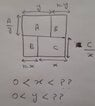# Finding minimum value of function with two variable

• MHB
gevni
I have a formula for cost calculation that contain x and y two variable. I have to find the value of (x,y) where that formula will gives minimum value as cost should not be equal to zero, it has some minimum value.
I took 1st partial derivative with respect to x and then with y and found the value of x and y where function will give minimum value. My question is how to combine both to get one answer as optimal (x,y) where function has minimum cost?

Last edited:

Gold Member
MHB

HOI
I have a formula for cost calculation that contain x and y two variable. I have to find the value of (x,y) where that formula will gives minimum value as cost should not be equal to zero, it has some minimum value.
I took 1st partial derivative with respect to x and then with y and found the value of x and y where function will give minimum value. My question is how to combine both to get one answer as optimal (x,y) where function has minimum cost?
I assume you set the partial derivatives to 0 and solved the two resulting equations for x and y. Now what do mean by "combine both to get one answer? The one point, (x, y) IS the point where f(x, y) is minimum (or maximum- you need to check which). For example if $f(x,y)= (x-3)^2+(y- 4)^2$ then setting the partial derivatives equal to 0 gives $2(x- 3)= 0$ and $2(y- 4)= 0$ so x= 3 and y= 4. The "critical point" is (3, 4) where the value of the function is f(3, 4)= 0, a minimum.

gevni
I assume you set the partial derivatives to 0 and solved the two resulting equations for x and y. Now what do mean by "combine both to get one answer? The one point, (x, y) IS the point where f(x, y) is minimum (or maximum- you need to check which). For example if $f(x,y)= (x-3)^2+(y- 4)^2$ then setting the partial derivatives equal to 0 gives $2(x- 3)= 0$ and $2(y- 4)= 0$ so x= 3 and y= 4. The "critical point" is (3, 4) where the value of the function is f(3, 4)= 0, a minimum.
Thank you! yes after getting partial derivatives with x and y I set the resulting equations to 0 for getting values of x and y and got the formula for x= ...and y=...
Just want to confirm that now I have to replace x and y in original cost equation with these formulas (x= and y=) and it will results in minimum cost for that cost equation right?.
One more question how do I know that these values of x and y are for minimum?

HOI
One way is to compare the value at that point to values close by. Another is to use the "second derivative test": if $(f_{xx})(f_{yy})-(f_{xy})^2$ is positive at the given point, then it is either a maximum or a minimum. If it is negative then the point is a "saddle point".

(This is the determinant of the "Hessian matrix", $\begin{bmatrix} f_{xx} & f_{xy} \\ f_{xy} & f_{yy}\end{bmatrix}$. It is actually the product of the two eigenvalues. Each eigenvalue tells you if the graph curves up (if the eigenvalue is positive) or down (if negative) in the x or y directions. The determinant is positive if they have the same sign, both positive or both negative, negative if they have different signs.)

Last edited:
gevni
One way is to compare the value at that point to values close by. Another is to use the "second derivative test": if $(f_{xx})(f_{yy})-(f_{xy})^2$ is positive at the given point, then it is either a maximum or a minimum. If it is negative then the point is a "saddle point".

Thank you I compared points closed by and get to know the minimum. Now there is one more thing, I have to provide the range of x and y within which if their values fall then there is a solution else there will be no solution. Can you please look into the attached figure it is n*n square divided into A,B and C area, can you please help me finding the upper range for both variables (x,y)?

0<x< ?
0<y<?

#### Attachments

•110461264_1177808379226535_2654335419636091325_n.jpg
38.5 KB · Views: 16
HOI
That picture is very hard to understand. You have region labeled "A", "B", "C", and "A" and are apparently using those letters to represent the areas of those regions but don't say that. In addition you have an "h" (or is it "n"?) but do not define it. Is h the length of a side of the square? Finally, what in the world does this picture have to do with the original problem, a "cost calculation"?

gevni
That picture is very hard to understand.
I think I batter had to ask it as a separate question (with detail) as it is a different problem. Many thanks for your help :)